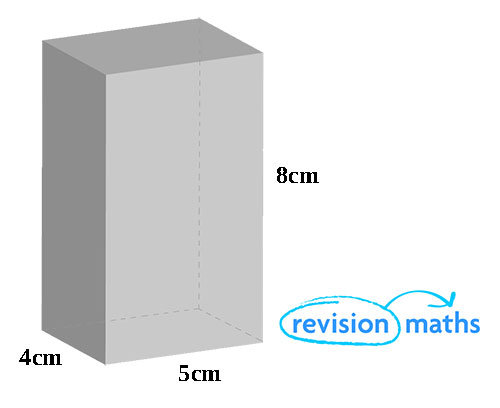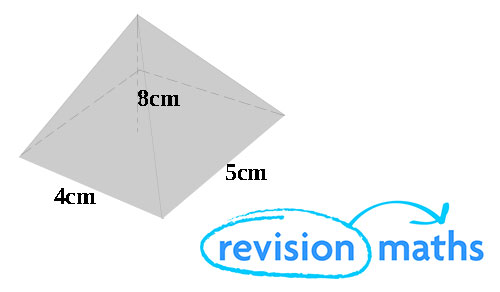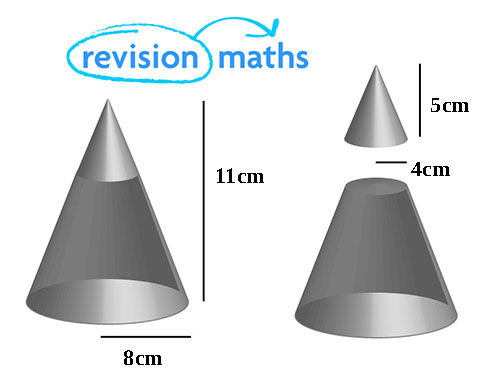## Volume

In this section of Revision Maths you will learn how to calculate the volume of prisms, pyramids and frustums.

Volume is a 3D Measurement so we need to always write answers as cubed numbers, for example CM3 or M3.

Volume of a Prism

To calculate the volume of a prism you need to use this formula: Area of a cross-section x height or length.In the example above the volume = 4 x 5 x8 = 160cm3

To find out how to work out the area of a 2D shape click here.

Volume of a Pyramid

To calculate the volume of a pyramid you use this formula: 1/3 area of a cross section x height.In the example above the volume = 1/3 x 4 x 5 x 8 = 53.33cm3 (to 4.s.f).

Volume of a Frustum

A frustum is made by cutting the top end of a cone to calculate the volume of a frustum use the formula below:

1/3 π R2 H – 1/3 πr2h

The second half of the formula is how to work out the volume of the entire cone.In the example above 1/3π [(82 x 11) – (42 x 5)

So the volume of this frustum = 208 πcm3 or 653cm3 (to 3 s.f)